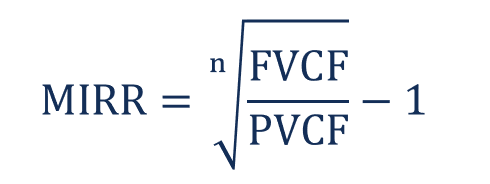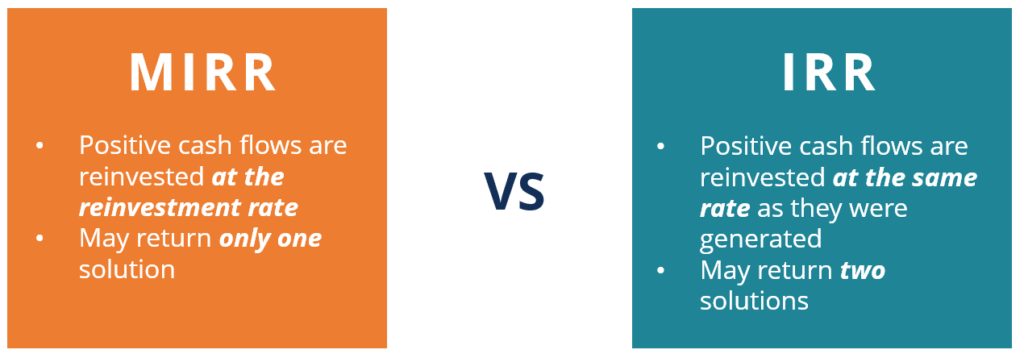# Modified Internal Rate of Return (MIRR)

A financial measure used in capital budgeting to identify the viability of an investment project

## What is the Modified Internal Rate of Return (MIRR)?

The modified internal rate of return (commonly denoted as MIRR) is a financial measure that determines the attractiveness of an investment, as well as it can be used to compare different investments. Essentially, the modified internal rate of return is a modification of the internal rate of return (IRR) formula, which resolves some issues associated with that financial measure.The MIRR is primarily used in capital budgeting to identify the viability of an investment project. For instance, if the MIRR of a project is higher than its expected return, an investment is considered to be attractive.

Conversely, it is not recommended to undertake a project if its MIRR is less than the expected return. In addition, the MIRR is commonly employed to compare several alternative projects that are mutually exclusive. In such a case, the project with the highest MIRR is the most attractive.

### How to Calculate the Modified Internal Rate of Return

Calculating the MIRR considers three key variables: (1) the future value of positive cash flows discounted at the reinvestment rate, (2) the present value of negative cash flows discounted at the financing rate, and (3) the number of periods.

Mathematically, the calculation of the MIRR is expressed using the following equation:Where:

• FVCF – the future value of positive cash flows discounted at the reinvestment rate
• PVCF – the present value of negative cash flows discounted at the financing rate
• n – the number of periods

Generally, the manual calculation of the MIRR is a tedious process that is prone to making mistakes. Alternatively, the MIRR can be easily calculated in spreadsheet applications such as Microsoft Excel. For example, in MS Excel, it can be calculated using the function called “=MIRR (cash flows, financing rate, reinvestment rate).”

### MIRR vs. IRR

The modified internal rate of return (MIRR) and the internal rate of return (IRR) are two closely-related concepts. The MIRR was introduced to address a few problems associated with the IRR. For example, one of the main problems with the IRR is the assumption that the obtained positive cash flows are reinvested at the same rate at which they were generated. Alternatively, the MIRR considers that the proceeds from the positive cash flows of a project will be reinvested at the external rate of return. Frequently, the external rate of return is set equal to the company’s cost of capital.

Also, in some cases, the calculations of IRR may provide two solutions. The fact creates ambiguity and unnecessary confusion regarding the correct outcome. Unlike the IRR, the MIRR calculations always return a single solution.

The common view is that the MIRR provides a more realistic picture of the return on the investment project relative to the standard IRR. Due to such a reason, the MIRR is commonly lower than the IRR.### Example of MIRR

Let’s consider the following example. Company A wants to assess the investment viability of its upcoming project of building a new plant. The company must spend \$200 million on the plant’s construction. At the same time, it expects that the new plant will generate revenues of \$50 million in the first year, \$100 million in the second year, and \$150 million in the third year. Note that the cost of capital of Company A is 10%.

Using the information above, we may calculate the modified internal rate of return of the project. Firstly, we need to calculate the future value of positive cash flows at the reinvestment rate. We may assume that the reinvestment rate equals the company’s cost of capital.

The present value of negative cash flows discounted at the financing rate is simply \$200 million because there is only one cash outflow occurring before the project. Therefore, we can use the variables to calculate the modified internal rate of return (MIRR):

The modified internal rate of return for the project is 17.02%. In order to determine the investment viability of the project, the figure may be later compared with the expected return of the project.

CFI is the official provider of the global Financial Modeling & Valuation Analyst (FMVA)™ certification program, designed to help anyone become a world-class financial analyst. To keep advancing your career, the additional resources below will be useful:

• Cash Flow Statement
• Due Diligence in Project Finance
• Return on Investment (ROI)
• Required Rate of Return

### Financial Analyst Training

Get world-class financial training with CFI’s online certified financial analyst training program!

Gain the confidence you need to move up the ladder in a high powered corporate finance career path.

Learn financial modeling and valuation in Excel the easy way, with step-by-step training.